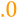# Round

 Block Group: Number Formatting Icon:The Round block rounds a number to a specified precision.

For information on using dataflow blocks, see Dataflow.

For accepted and excluded arguments of Number Formatting blocks, see Number Formatting.

## Input/Output Properties

The following properties of the Round block can take input and give output.

• input (number)
• precision (number)

input specifies any real number that you want to round.

precision specifies the number of digits after the decimal to round the number to. A value of 0, less than 0, or null rounds the number to the nearest integer.

## Output Property

The following property of the Round block can give output but cannot take input.

• output (number)

output returns the rounded number.

## Examples

The following image shows an example of the Round block. In this example, pi is rounded to a precision of three digits.